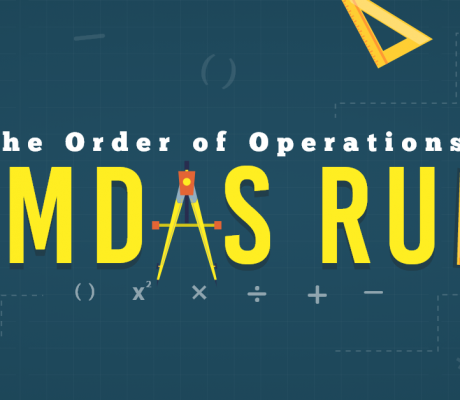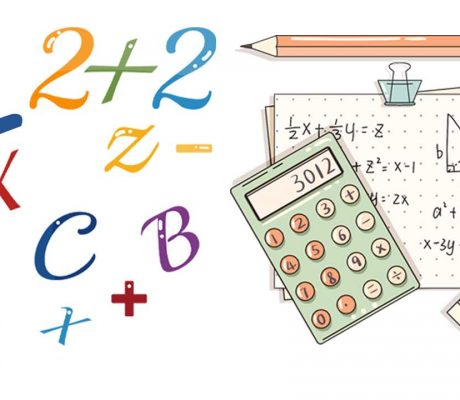# Category: Subtraction### The Order of Operations: PEMDAS Rule

In Japan, the math problem went viral after a study found that only 60% of individuals in their 20’s were able to get the correct answer, lower than the 90% success rate in the 1980s. This study reflects the reality around the world that more and more students have given less importance to mastering the […]### Equivalent Fractions

Equivalent fractions are fractions that represent the same size even if the numbers look different. Let’s take a look at the pizzas below. The two fractions have different numerators and denominators, but they represent the same size of pizza slices. when simplified, is actually . It is important to remember that these two fractions still […]### Learn ZOE Math Practice Questions with Answers

In this blog, there are 21 math practice questions that Learn ZOE teachers prepared for you. The questions are based on different grade levels and once the answer is revealed, there will be a detailed explanation of how we got the correct answer. Feel free to answer the math practice questions first before revealing the […]### Adding and Subtracting Fractions

Adding and Subtracting fractions may seem a terrifying Math lesson for some, but the truth is it is not as complicated as some people might think. The trick is to be able to recognize and translate fractions into familiar objects; which is why pizza slices and chocolate bars are commonly used to represent fractions. This […]